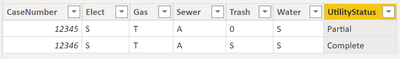cancel
Showing results for
Did you mean:Anonymous
Not applicable

## Generate new column based on row values

I have a table with the following columns

CaseNumber    Elect    Gas    Sewer   Trash  Water

Elect, Gas, Sewer, Trash, and Water; they all have 0 as the default value. Each CaseNumber has these 5 fields.

Example:

CaseNumber    Elect    Gas    Sewer   Trash  Water

12345                 S          T          A          0        S

12346                 S          T          A          S        S

I want a formula that returns a column (UtilityStatus) if the following conditions are met:

Pseudocode:

If(Elect,Gas, Sewer, Trash & Water are all diff from 0, Complete, Partial)

Therefore, following examples above, this is the result I shoul get.

CaseNumber    Elect    Gas    Sewer   Trash  Water  UtilityStatus

12345                 S          T          A          0        S          Partial

12346                 S          T          A          S        S          Complete

1 ACCEPTED SOLUTIONSuper User

Hi @Anonymous ,Here the DAX for the calculated coumn =

```UtilityStatus =
SWITCH (
TRUE(),
Table[Elect] = "0", "Partial",
Table[Gas]   = "0", "Partial",
Table[Sewer] = "0", "Partial",
Table[Trash] = "0", "Partial",
Table[Water] = "0", "Partial",
"Complete"
)```

My first question was, what you wanna return if all columns are 0, but that might not even happen in your data. So, I was just stubbornly following your requirement 🙂

Let me know if this helps 🙂

Proud to be a Super User!

2 REPLIES 2Super User

Hi @Anonymous ,Here the DAX for the calculated coumn =

```UtilityStatus =
SWITCH (
TRUE(),
Table[Elect] = "0", "Partial",
Table[Gas]   = "0", "Partial",
Table[Sewer] = "0", "Partial",
Table[Trash] = "0", "Partial",
Table[Water] = "0", "Partial",
"Complete"
)```

My first question was, what you wanna return if all columns are 0, but that might not even happen in your data. So, I was just stubbornly following your requirement 🙂

Let me know if this helps 🙂

Proud to be a Super User!Anonymous
Not applicable

This worked perfectly.

Answering your question: if all my columns are 0, I want "Partial", which of course happens with your formula. Thank you!